# MRO了解：

对于支持继承的编程语言来说，其方法（属性）可能定义在当前类，也可能来自于基类，所以在方法调用时就需要对当前类和基类进行搜索以确定方法所在的位置。而搜索的顺序就是所谓的「方法解析顺序」（Method Resolution Order，或MRO）。对于只支持单继承的语言来说，MRO 一般比较简单；而对于 Python 这种支持多继承的语言来说，MRO 就复杂很多。

# 而具体讨论MRO，我们需要针对不同python版本中的MRO进行解析

经典类：DFS深度优先搜索（Python2.2以前的版本）

## 一：经典类（深度优先搜索）

在经典类中，没有__mro__属性可以去查看MRO的顺序，但是，可以使用inspect模块中getmro方法

import inspect
inspect.getmro(类名)

### （一）正常继承模式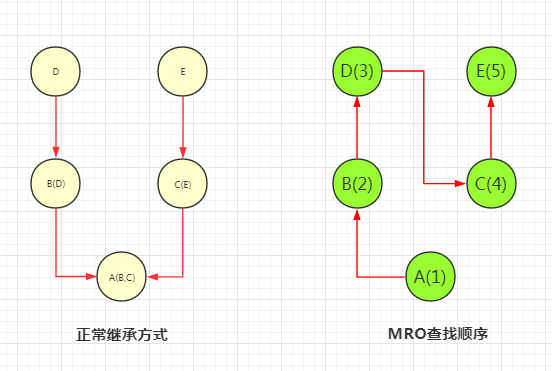### （二）交叉继承模式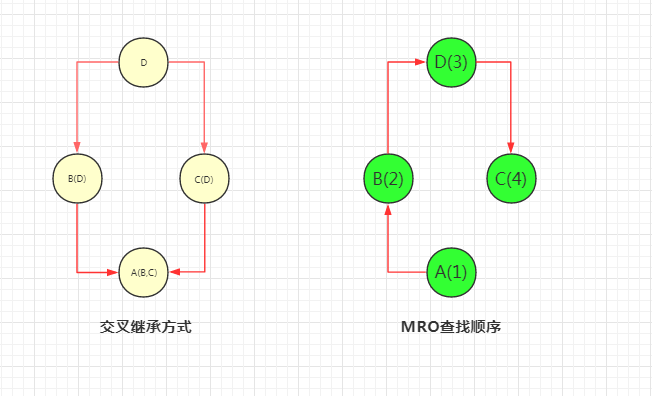import inspect

class D:
def foo(self):
print("D.foo")

class C(D):
def foo(self):
print("C.foo")

class B(D):
pass

class A(B,C):
pass

print(inspect.getmro(A))    #A->B->D->C
obj = A()
obj.foo()   #D.foo

## 二：新式类（广度优先搜索）

### （一）正常继承方式class E(object):
pass

class D(object):
def foo(self):
print("D.foo")

class C(E):
def foo(self):
print("C.foo")

class B(D):
pass

class A(B,C):
pass

print(A.__mro__)    #A->B->C->D->E
obj = A()
obj.foo()   #C.foo

### （二）交叉继承方式## 三：新式类（C3算法实现：看起来就是将上面两者的优点结合了。所以在Python3中全部都是新式类，不需要object继承）

### （一）正常继承方式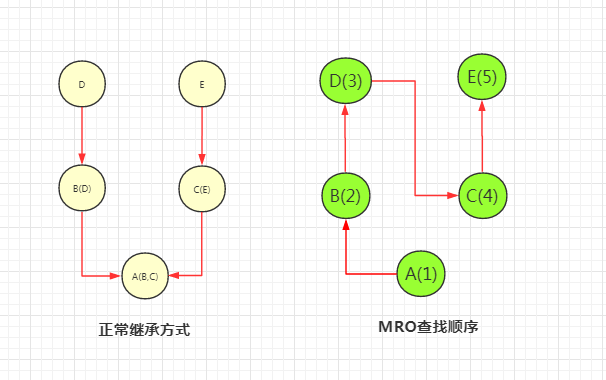### （二）交叉继承方式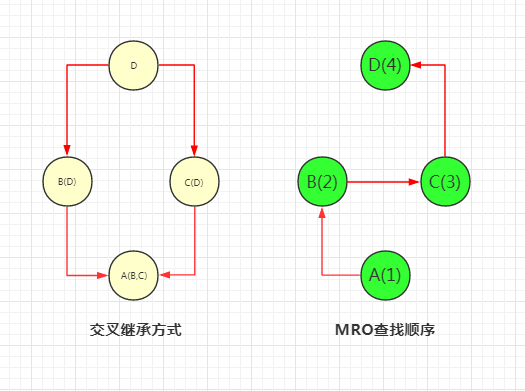在python3中这种MRO方法是唯一使用的。

## 四：C3算法了解

推文：C3算法了解

推文：C3算法了解（这个更加详细）

注意：我们把类 C 的线性化（MRO）记为 L[C] = [C1, C2,…,CN]。其中 C1 称为 L[C] 的头，其余元素 [C2,…,CN] 称为尾
L[object] = [object]
L[C(B1,B2,...,B(N-1),BN)] = [C] + merge(L[B1]+L[B2]+...+L[B(N-1)]+L[BN], [B1,B2,...,B(N-1),BN]) #这种解法是正确的
其他地方可以看上面两篇推文即可（第二篇更加详细）

### 步骤：

1.检查第一个列表的头元素（如 L[B1] 的头），记作 H。
2.若 H 未出现在其它列表的尾部，则将其输出，并将其从所有列表中删除，然后回到步骤1；否则，取出下一个列表的头部记作 H，继续该步骤。（重点）
3.重复上述步骤，直至列表为空或者不能再找出可以输出的元素。如果是前一种情况，则算法结束；如果是后一种情况，说明无法构建继承关系，Python 会抛出异常。

### （一）推文一：案例推导（其中o代表object类）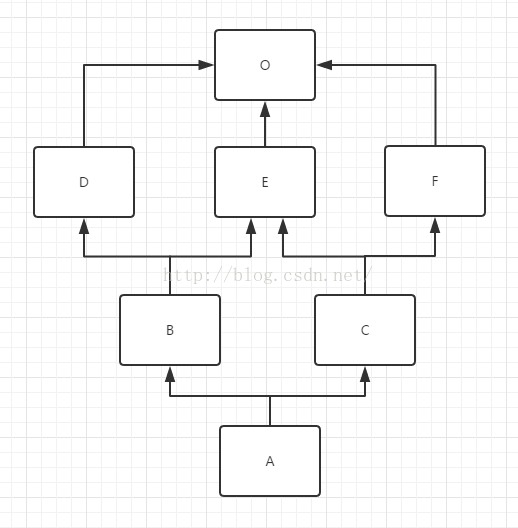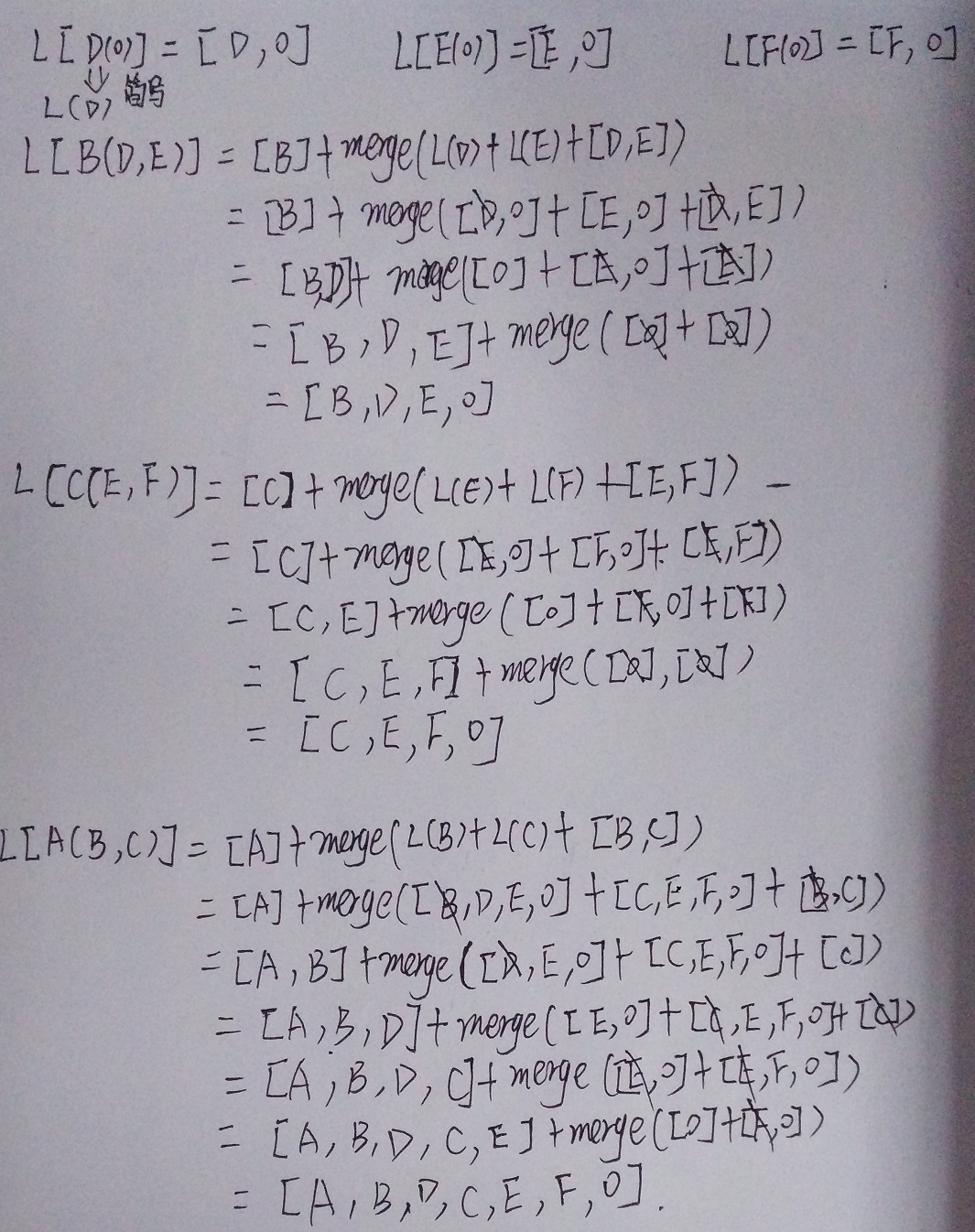### （二）推文二：案例推导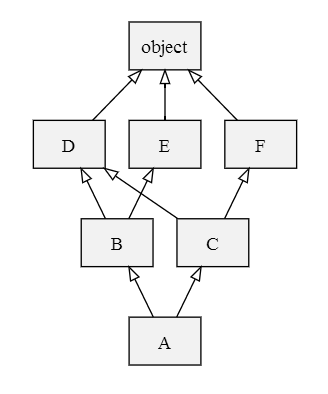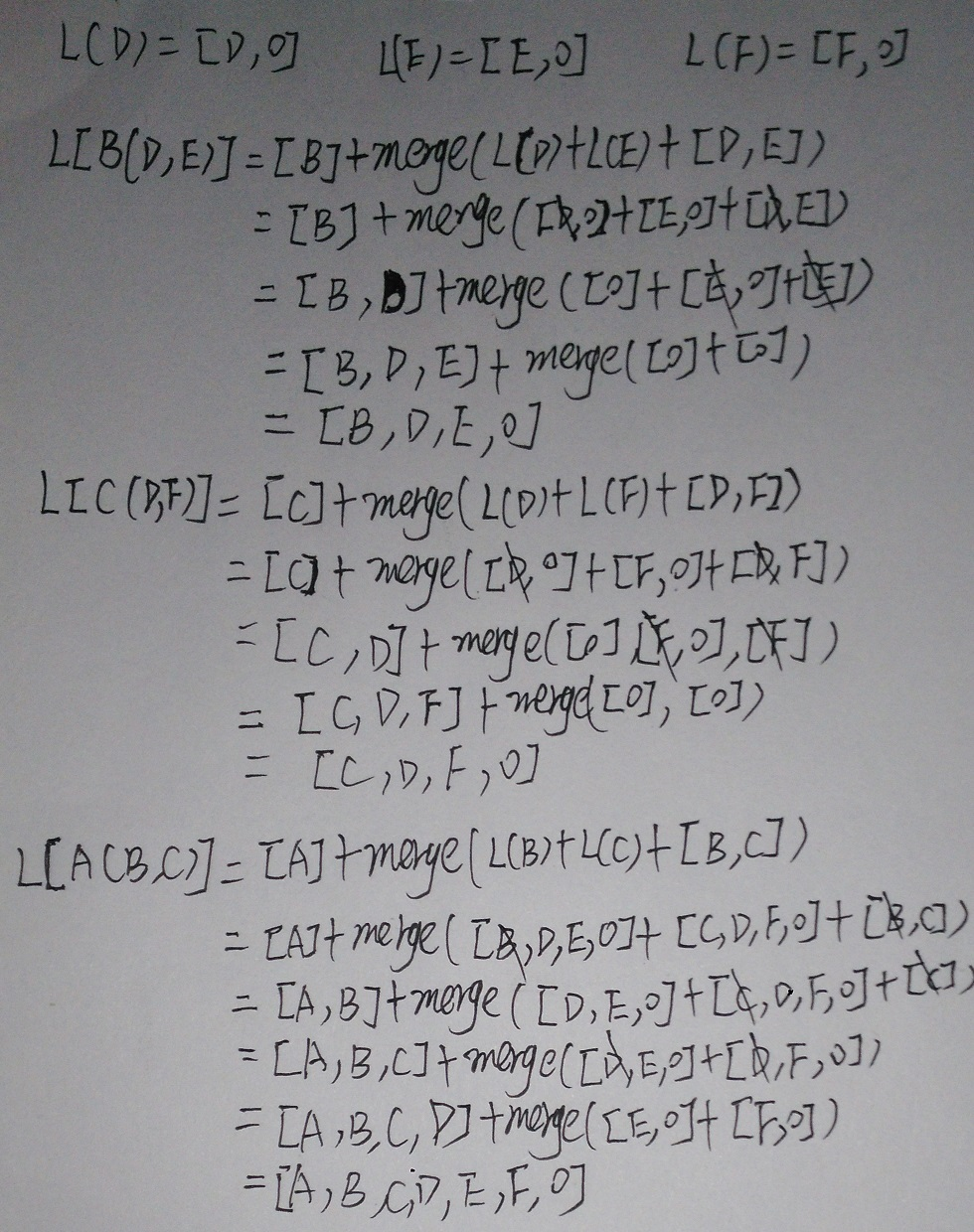# 下面讨论__init__和super()方法的关系

## 一：在单继承中super方法和__init__使用时功能上基本是无差别的

class A(object):
def __init__(self):
print("A.__init__.start")
print("A.__init__.end")

class B(A):
def __init__(self):
print("B.__init__.start")
super(B, self).__init__()
print("B.__init__.end")

class C(A):
def __init__(self):
print("B.__init__.start")
A.__init__(self)
print("B.__init__.end")

b = B()
# B.__init__.start
# A.__init__.start
# A.__init__.end
# B.__init__.end

c = C()
# B.__init__.start
# A.__init__.start
# A.__init__.end
# B.__init__.end

## 二：super方法只在新式类中适用

>>> class A:
...     def __init__(self):
...         print("A.__init__.start")
...         print("A.__init__.end")
...
>>> class B:
...     def __init__(self):
...         print("B.__init__.start")
...         super(B, self).__init__()
...         print("B.__init__.end")
...
>>> class C(A):
...     def __init__(self):
...         print("B.__init__.start")
...         A.__init__(self)
...         print("B.__init__.end")
...
>>> b = B()
B.__init__.start
Traceback (most recent call last):
File "<stdin>", line 1, in <module>
File "<stdin>", line 4, in __init__
TypeError: must be type, not classobj

## 三：注意super()不是父类，而是执行MRO顺序中的下一个类！！

class E(object):
def __init__(self):
print("E")

class D(object):
def __init__(self):
print("D")
super(D, self).__init__()

class C(E):
def __init__(self):
print("C")
super(C, self).__init__()

class B(D):
def __init__(self):
print("B")
super(B, self).__init__()

class A(B,C):
def __init__(self):
print("A")
super(A, self).__init__()

print(A.__mro__)    #A->B->D->C->E
obj = A()   #ABDCE

def super(class_name, self):
mro = self.__class__.mro() #获取mro的列表
return mro[mro.index(class_name) + 1]　　#获取自己的索引号，去返回下一个类

## 四：super()可以避免重复调用

### （一）__init__方法可能导致被执行多次

class A(object):
def __init__(self):
print("A.__init__")

class B(A):
def __init__(self):
print("B.__init__")
A.__init__(self)

class C(B,A):
def __init__(self):
print("C.__init__")
A.__init__(self)
B.__init__(self)

c = C()
C.__init__
A.__init__
B.__init__
A.__init__　　#出现重复调用

class C(A,B):　　#会因为无法创建MRO而出错
def __init__(self):
print("C.__init__")
A.__init__(self)
B.__init__(self)

TypeError: Cannot create a consistent method resolution
order (MRO) for bases A, B

### （二）使用super方法可以避免重复调用

class A(object):
def __init__(self):
print("A.__init__")

class B(A):
def __init__(self):
print("B.__init__")
super(B, self).__init__()

class C(B,A):
def __init__(self):
print("C.__init__")
super(C, self).__init__()

c = C()
C.__init__
B.__init__
A.__init__

class C(A,B):　　#也是因为无法生成MRO顺序出错
def __init__(self):
print("C.__init__")
super(C, self).__init__()

TypeError: Cannot create a consistent method resolution
order (MRO) for bases A, B

### 详细解决可以在推文：C3算法了解（2）中看到。下面也会写产生的原因（在生成MRO顺序时出错）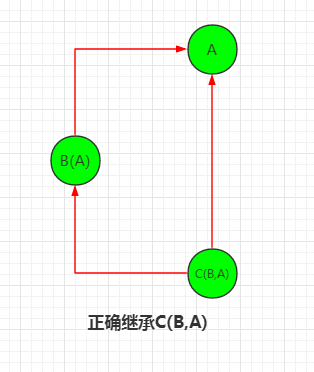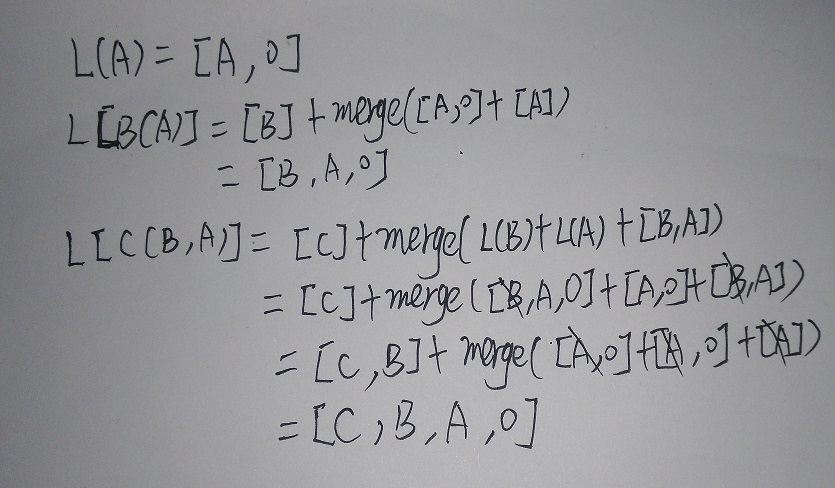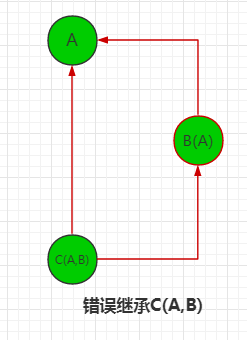C3算法解题：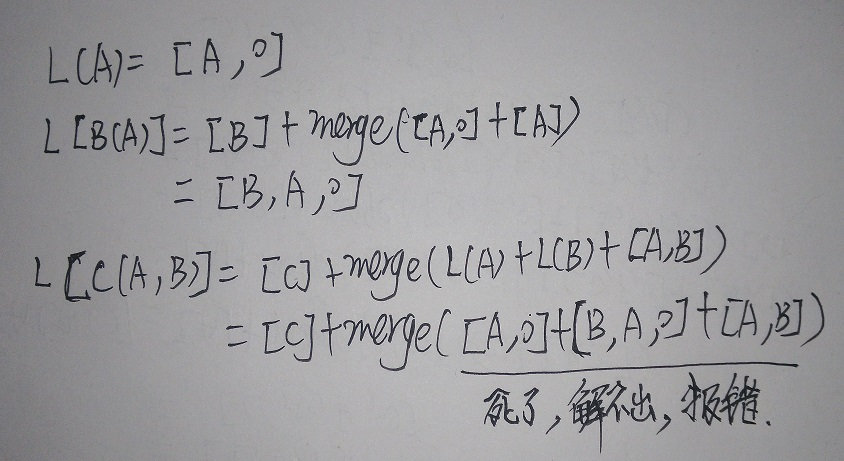posted @ 2018-05-09 23:18  山上有风景  阅读(2194)  评论(1编辑  收藏  举报